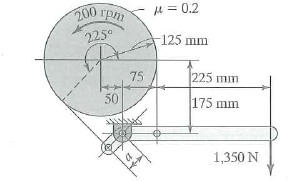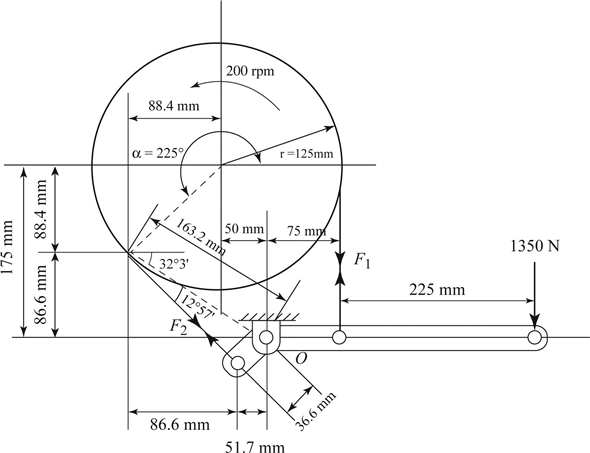Problem

# Find the horsepower that the brake of Fig. 1 can absorb, and the length of arm a.Figure 1

Find the horsepower that the brake of Fig. 1 can absorb, and the length of arm a.

Figure 1#### Step-by-Step Solution

Solution 1

Sketch the free body diagram for the brake as in Figure (1).Refer to Figure (1):

Length of the arm is 36.6 mm.

Express friction power for the brake as:

$$P=\frac{T n}{9,550,000} \ldots \ldots \text { (1) }$$

Here, friction power for the brake is $$P$$, torque exerted by the brake is $$T$$, and speed is $$n$$.

To find power, calculate the torque first:

From Figure (1), torque is expressed as:

$$T=\left(F_{1}-F_{2}\right) r \ldots \ldots \text { (2) }$$

Here, tension exerted at point 1 is $$F_{1}$$, tension exerted at point 2 is $$F_{2}$$, and drum radius is $$r$$.

Find the torque, first calculate the tension involved.

Apply static moment of equilibrium about point $$A$$.

\begin{aligned} &(1350 \mathrm{~N})(300 \mathrm{~mm})+\left(F_{2}\right)(36.6 \mathrm{~mm})-\left(F_{1}\right)(75 \mathrm{~mm})=0 \\ &405,000+36.6 F_{2}-75 F_{1}=0 \end{aligned}

Express tensions in band brake along with angle of contact as:

$$\frac{F_{1}}{F_{2}}=e^{j u}$$

Here, tension exerted at point 1 is $$F_{1}$$, tension exerted at point 2 is $$F_{2}$$, coefficient of friction is $$\mu$$, and angle of contact is $$\alpha$$.

Substitute $$0.15$$ for $$\mu$$ and $$210^{\circ}$$ for $$\alpha$$.

\begin{aligned} &\frac{F_{1}}{F_{2}}=e^{(02)(225)\left(\frac{\pi}{180^{\circ}}\right)} \\ &F_{1}=e^{0.7854} F_{2} \\ &F_{1}=2.193 F_{2} \end{aligned}

Substitute Equation (4) in Equation (3) to find $$F_{2}$$.

\begin{aligned} &405,000+36.6 F_{2}-75\left(2.193 F_{2}\right)=0 \\ &127.875 F_{2}=405,000 \\ &F_{2}=\frac{405,000}{127.875} \\ &F_{2}=3,167 \mathrm{~N} \end{aligned}

Substitute $$3,167 \mathrm{~N}$$ for $$F_{2}$$ in Equation (4) to find $$F_{1}$$.

\begin{aligned} F_{1} &=2.193(3,167 \mathrm{~N}) \\ &=6,945 \mathrm{~N} \end{aligned}

Calculate the torque exerted by the brake using Equation (2).

Substitute 6,945 $$\mathrm{N}$$ for $$F_{1}, 3,167 \mathrm{~N}$$ for $$F_{2}$$, and $$125 \mathrm{~mm}$$ for $$r$$ in Equation (2) to find $$T$$.

\begin{aligned} T &=(6,945 \mathrm{~N}-3,167 \mathrm{~N})(125 \mathrm{~mm}) \\ &=(3,778)(125) \\ &=472,250 \mathrm{~N} \mathrm{~mm} \end{aligned}

Calculate the friction power using Equation (1).

Substitute $$472,250 \mathrm{~N} \mathrm{~mm}$$ for $$T$$ and $$200 \mathrm{rpm}$$ for $$n$$ in Equation (1) to find $$P$$.

\begin{aligned} P &=\frac{(472,250 \mathrm{~N} \mathrm{~mm})(200 \mathrm{rpm})}{9,550,000} \\ &=9.89 \mathrm{~kW} \end{aligned}